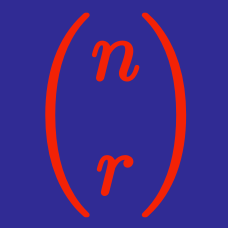Probability

# Binomial Theorem - N Choose K

Evaluate ${42 \choose 40}.$

What value of $n$ satisfies ${n \choose 2}=465?$

What is the coefficient of $x^ 5$ in the expansion of $(x+1)^ {8}$?

What is the value of positive integer $n$ satisfying ${22 \choose n}={22 \choose {n-10}}?$

What is the coefficient of the $x^ 4 y^ 4$ term in the expansion of $(x+y)^{8}$?

×